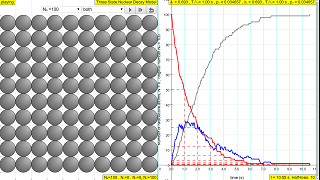### Translations

Code Language Translator Run### Software Requirements

SoftwareRequirements

 Android iOS Windows MacOS with best with Chrome Chrome Chrome Chrome support full-screen? Yes. Chrome/Opera No. Firefox/ Samsung Internet Not yet Yes Yes cannot work on some mobile browser that don't understand JavaScript such as..... cannot work on Internet Explorer 9 and below

### CreditsFu-Kwun Hwang - Dept. of Physics, National Taiwan Normal Univ.; lookang

### end faq

http://iwant2study.org/lookangejss/06QuantumPhysics_20nuclear/ejss_model_decaychangeNweethreestate/decaychangeNweethreestate_Simulation.xhtml

### Theory

The radioactive nuclei RED will decay into daughter product, and the daughter nucleus will decay grand-daughter stable nucleus.
The original nuclei are color code in Red
It will decay into blue-> grey (and become stable nuclei).

You can change decay half life time (unit: second) for each stage  (from red-> blue -> grey),
click start and watch how the number of different nuclei changed with time -- colored traces.

The law of radioactive decay predicts how the number of the not decayed nuclei of a given radioactive substance decreases in the course of time. The RED circles of this simulation symbolize 100 (variable selectable up 400 or 1024) to  atomic nuclei of a radioactive substance whose half-life period (T1 1/2) amounts to 0.1 to 3.0 seconds. However, in real life, sometimes these intermediate state N2  in BLUE are unstable themselves and are also radioactive whose half-life period (T1/2) amounts to 0.1 to 3.0 seconds, into the stable state N3 GREY.

The scientfic graph on the right, in number of radioactive atoms versus time, RED trail represents represents the Number of radioactive atoms, N₁ not yet decayed nuclei at a given time t, predicted by the following law:

N1   =   N0 e -(ln2/T1/2)) t

when you play with the show model option, show me N1= No*exp(-ln(2)/T1/2*t)

the terms use are

N1 .... number of the not decayed nuclei

N2 .... number of the decayed nuclei

N0 ... number of the initially existing nuclei at time, t = 0
t .... time
T1/2 ....  is the time taken for the activity of a given amount of a radioactive substance to decay to half of its initial value

λ,  or sometimes also known as "lambda" the inverse of the mean lifetime, sometimes referred to as simply decay rate.

p  is probability of decaying where 0 means no chance at all, 1 is 100% chance of decaying

Formula that related some of these terms are

T1/2 = ln(2)/λ

$$\lambda = \frac{p}{dt}$$

The Ordinary Differential equations used is

A three-state radioactive sample. Radionuclides change from red to green to blue as they decay. If the number of nuclides is large, the upper state N1, intermediate state N2, and final state N3 populations obey the following coupled ordinary differential equations (ODEs)

$$\frac{dN_{1}}{dt} = - \lambda_{1}N_{1}$$

$$\frac{dN_{2}}{dt} = + \lambda_{1}N_{1} - \lambda_{2}N_{2}$$

$$\frac{dN_{3}}{dt} = - \lambda_{2}N_{2}$$

where λ1 is the upper state decay rate and λ2 is the intermediate state decay rate. The nature of the decay is governed by these decay constants

### Other Resources

2. https://iwant2study.org/taskmeister/index.php/interactive-resources/physics/06-quantum-physics/02-nuclear/315-decaychangenwee by Fu-Kwun Hwang and lookang JavaScript version with only 2 states.
3. http://www.opensourcephysics.org/items/detail.cfm?ID=10577 by Wolfgang Christian Java version similar in idea
4. http://www.phy.ntnu.edu.tw/ntnujava/index.php?topic=194.0 by Fu-Kwun Hwang 2 state Java version

# Testimonials (0)

There are no testimonials available for viewing. Login to deploy the article and be the first to submit your review!